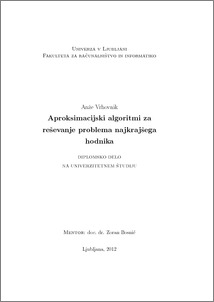# Approximation algorithms for solving the minimal corridor problem

Anže Vrhovnik (2012) Approximation algorithms for solving the minimal corridor problem. EngD thesis.Preview
PDF

## Abstract

In the thesis we will discuss the minimum-length corridor problem. Given a rectangular boundary partitioned into rectangles, the problem is to find a minimum edge-length corridor that visits at least one point from the boundary of every rectangle and from the rectangular boundary. We develop three approximation algorithms to solve this problem and compare them to the algorithm which always finds the optimal corridor. With the first algorithm, we try to build a corridor with the shortest walls only. The second algorithm adds the best walls to the corridor, until we get the acceptable solution. With the third algorithm, we value the vertexes and add the best of them to the corridor, until we achieve the acceptable result. The results show that the use of approximation algorithms considerably shortens the search time but gives us slightly worse results. Using approximation algorithms is recommended for larger problems since the waiting time for calculation of optimum corridor would take too long.

Item Type: Thesis (EngD thesis)
Keywords: minimum-length corridor problem, approximation algorithm, shortest path in a graph
Number of Pages: 48
Language of Content: Slovenian
Mentor / Comentors:
Name and SurnameIDFunction
doc. dr. Zoran Bosnić3826Mentor